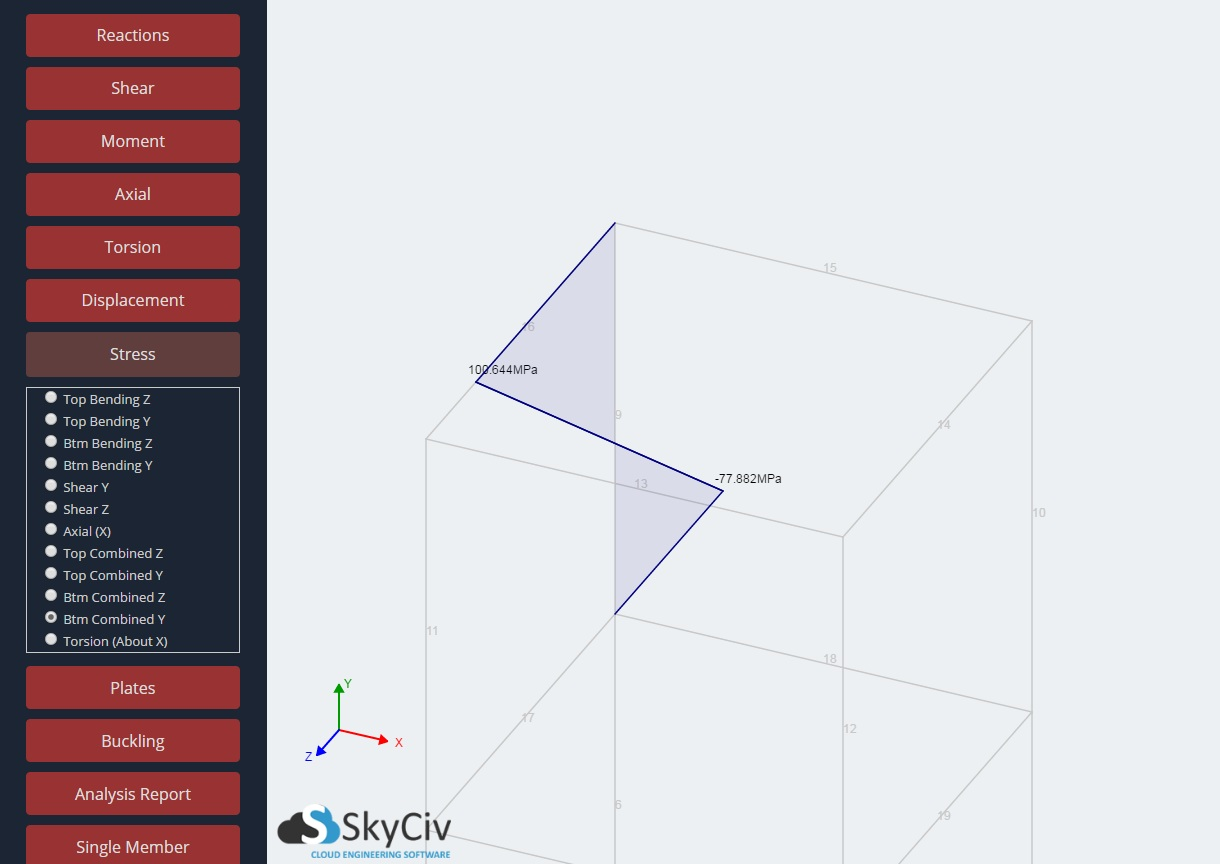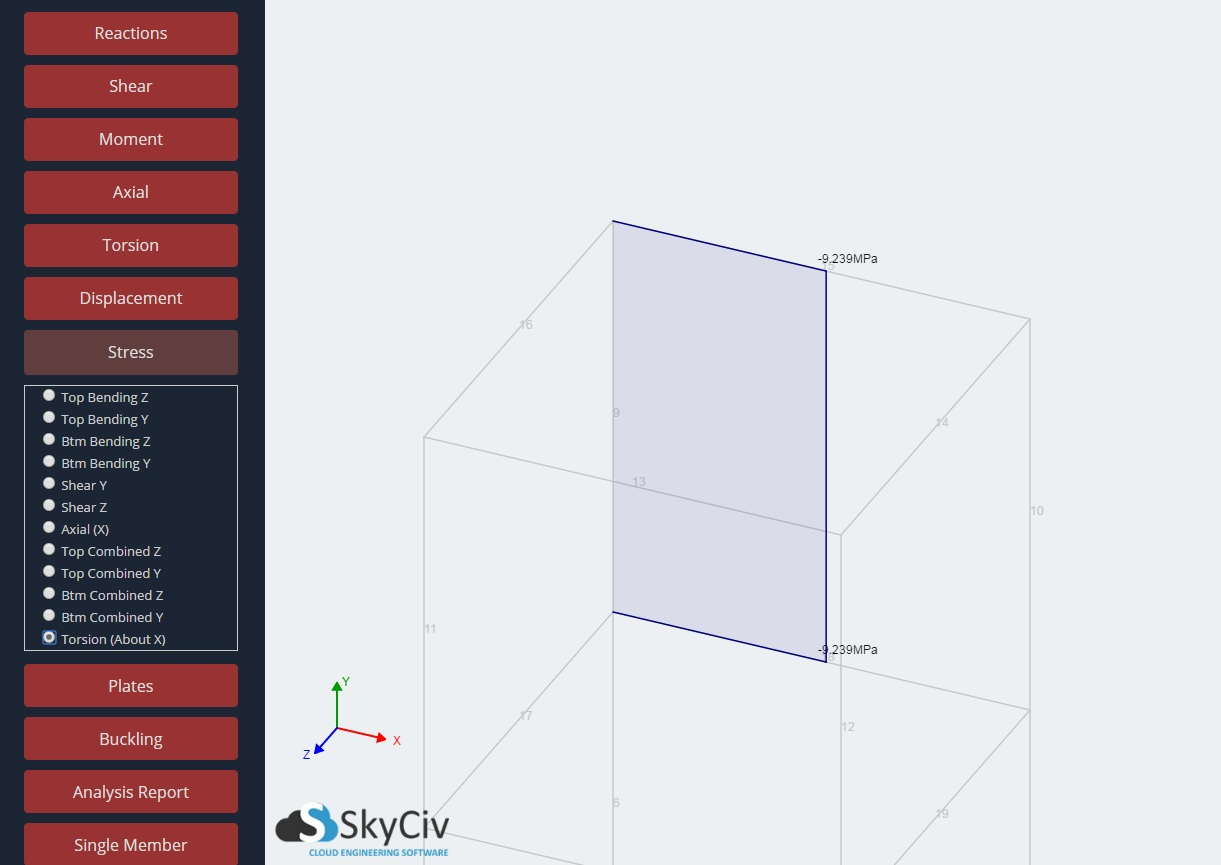Documentation [Deprecated]
SkyCiv Documentation

Getting Started

Section Builder
Operations
Built-Up and Composite Sections
Custom Shapes

Member Design
General
Member Design Modules
Code Verification

RC Design
General
Code Verification

# Stress

Display the single member stress values acting on each member of your structure. The stress types that can be viewed are separated based on their component local axis directions and include:

• Top Bending Z
• Top Bending Y
• Btm Bending Z
• Btm Bending Y
• Shear Y
• Shear Z
• Axial (X)
• Top Combined Z
• Top Combined Y
• Btm Combined Z
• Btm Combined Y

## Local Axes

The diagram below explains how local axes are described for each member to better visualise how stress results are represented.

• Local X axis - In the direction along the length of the member (longitude axis).
• Local Y axis - In the direction of the altitude axis. The vertical axis of the cross section.
• Local Z axis - In the direction of the lateral axis. The horizontal axis of the cross section.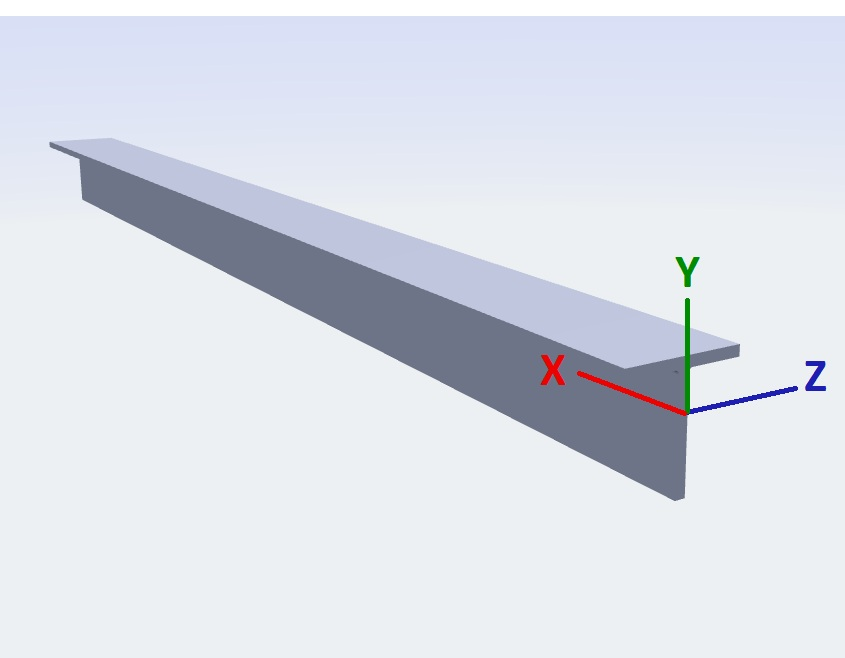## Top Bending Z

The top fibre bending stress about the local Z axis. Acts in the local X axis.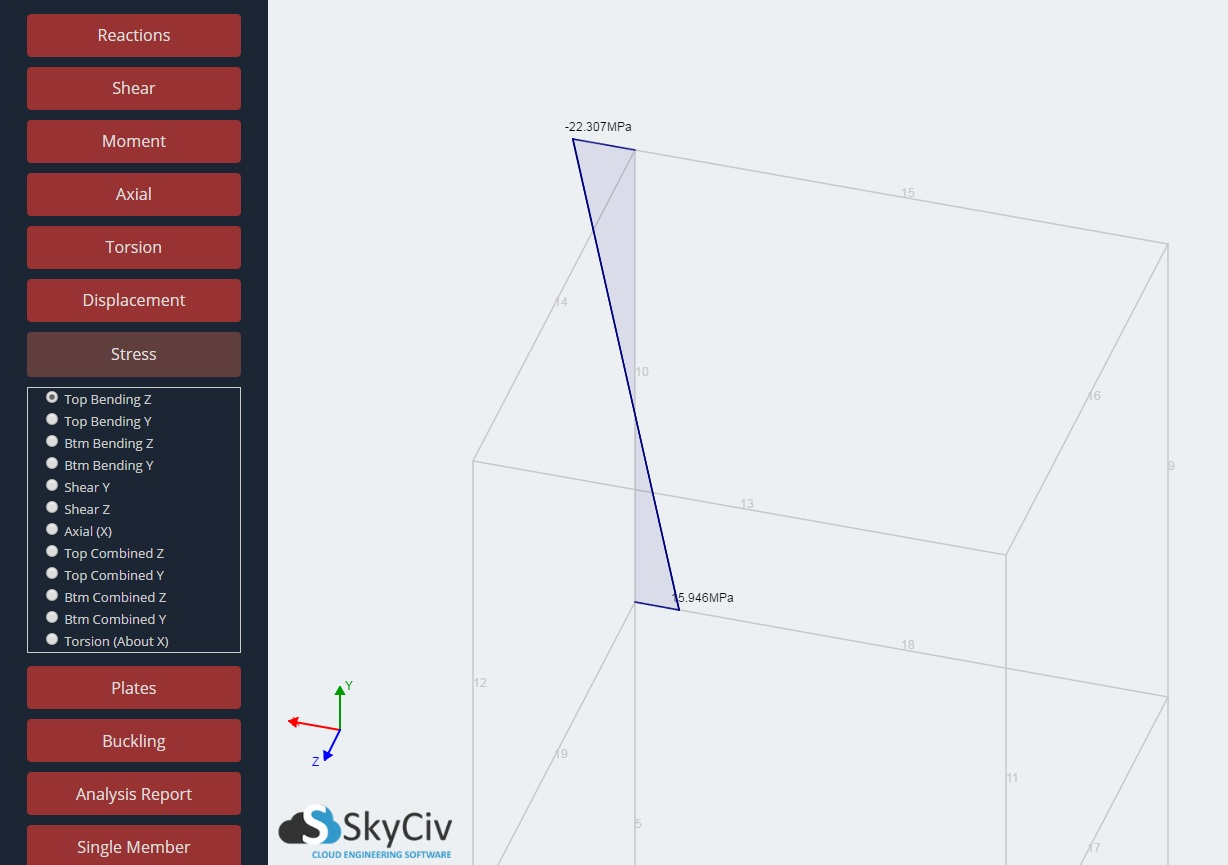## Top Bending Y

The top fibre bending stress about the local Y axis. Acts in the local X axis.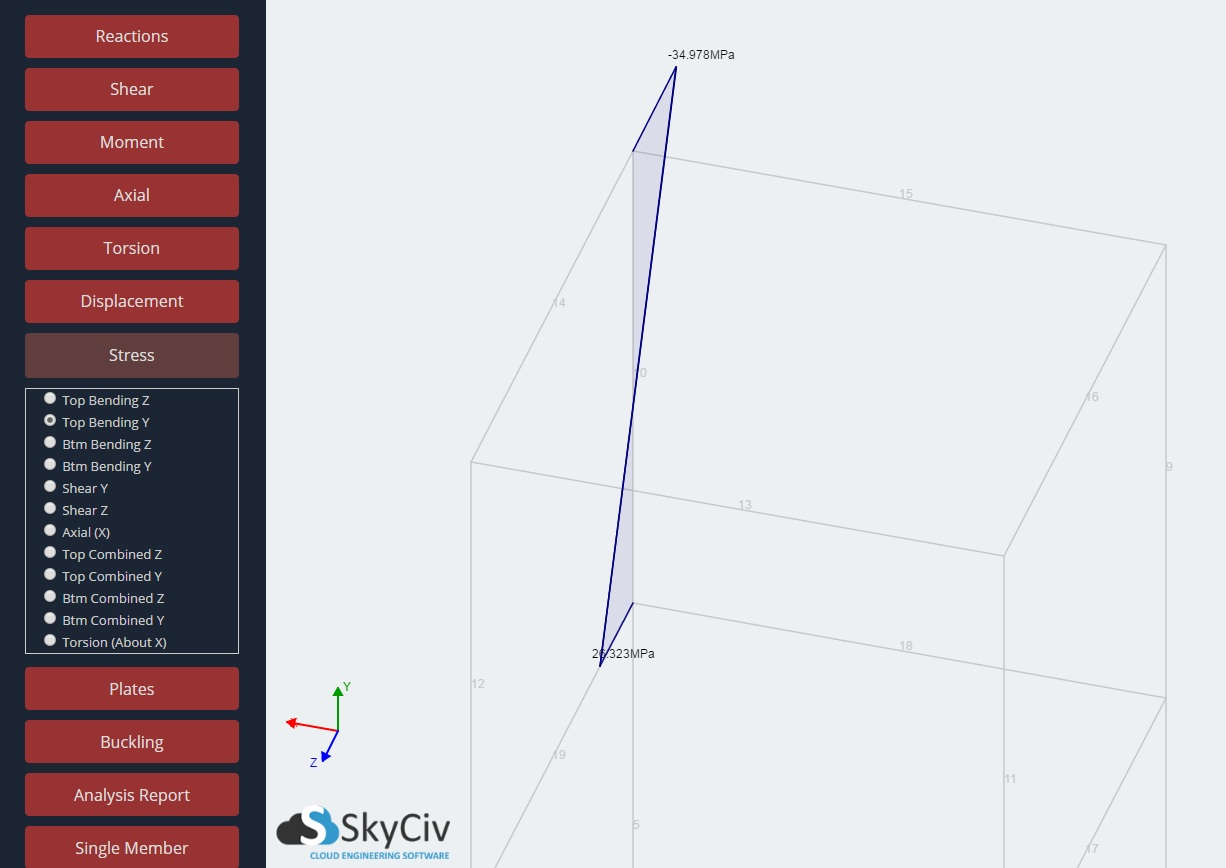## Bottom Bending Z

The bottom fibre bending stress about the local Z axis. Acts in the local X axis.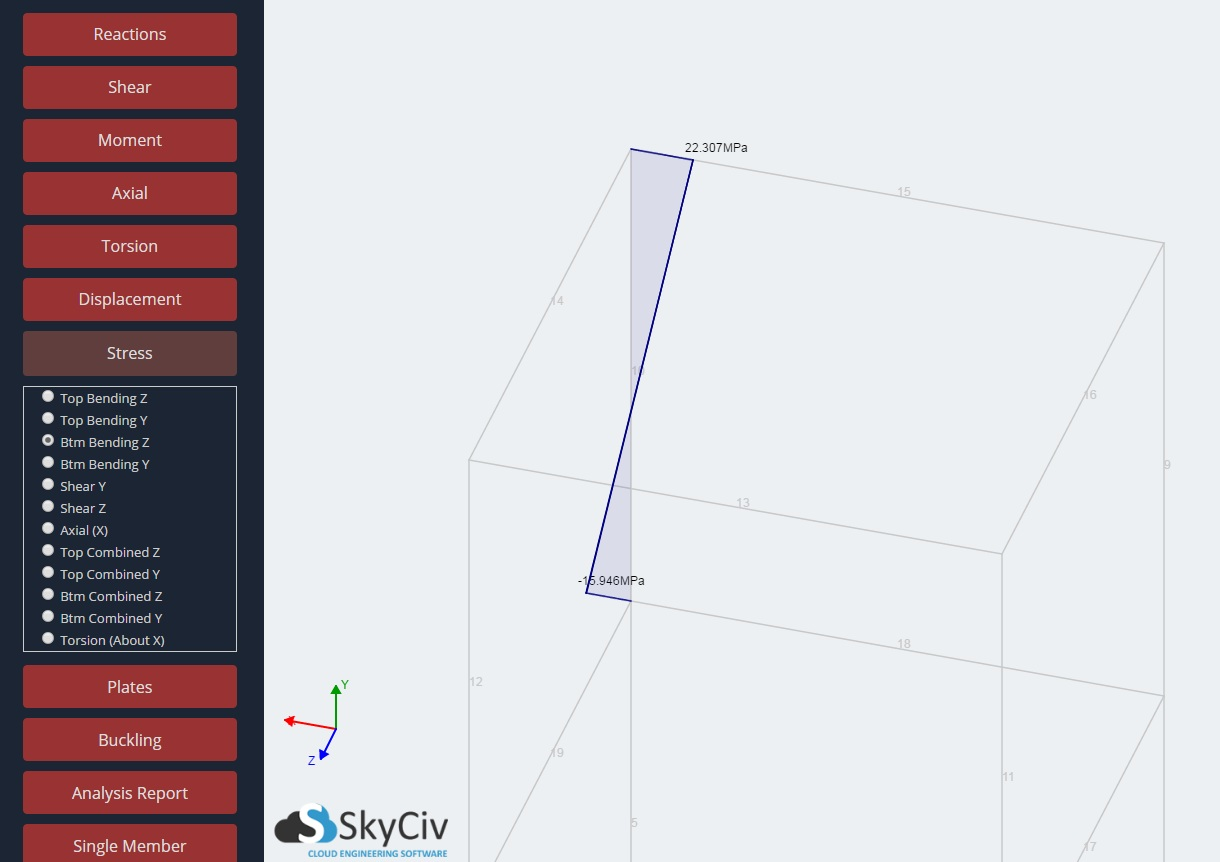## Bottom Bending Y

The bottom fibre bending stress about the local Y axis. Acts in the local X axis.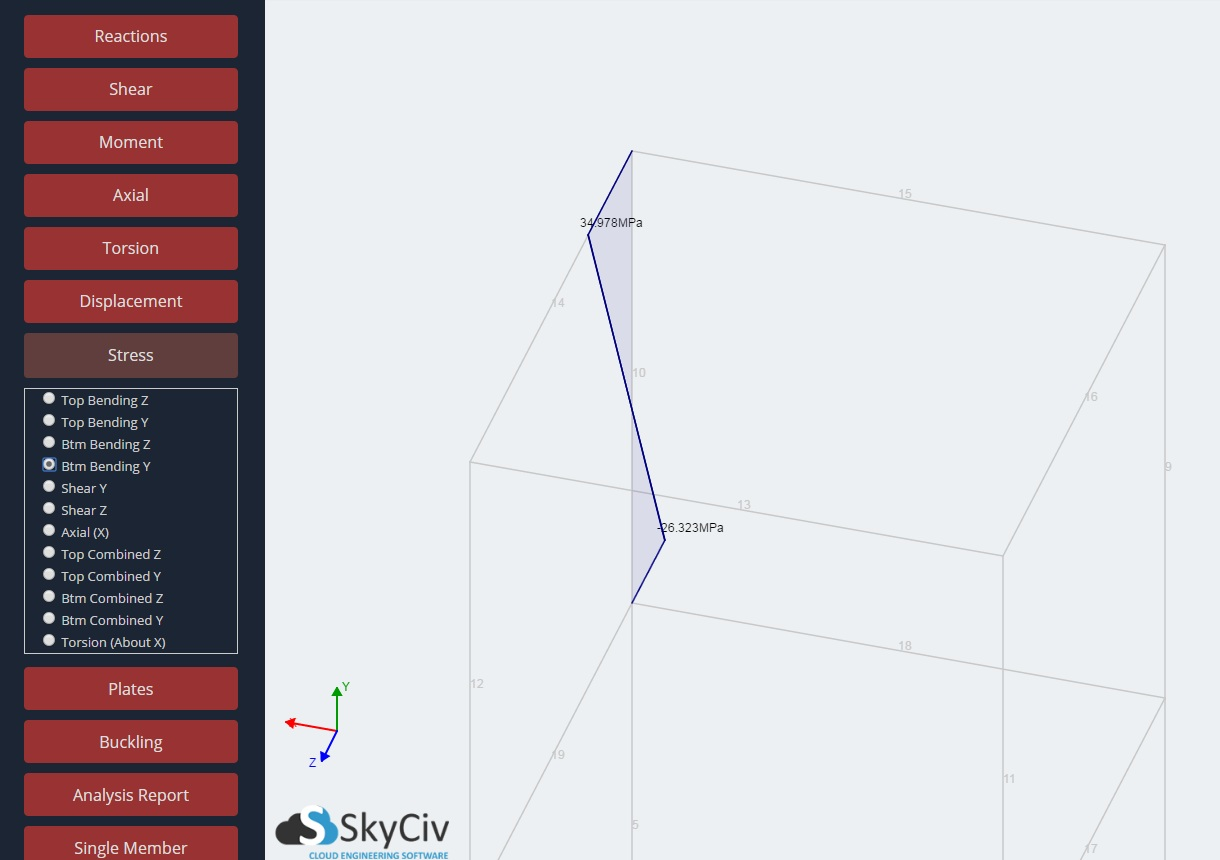## Shear Y

The shear stress in the local Y axis.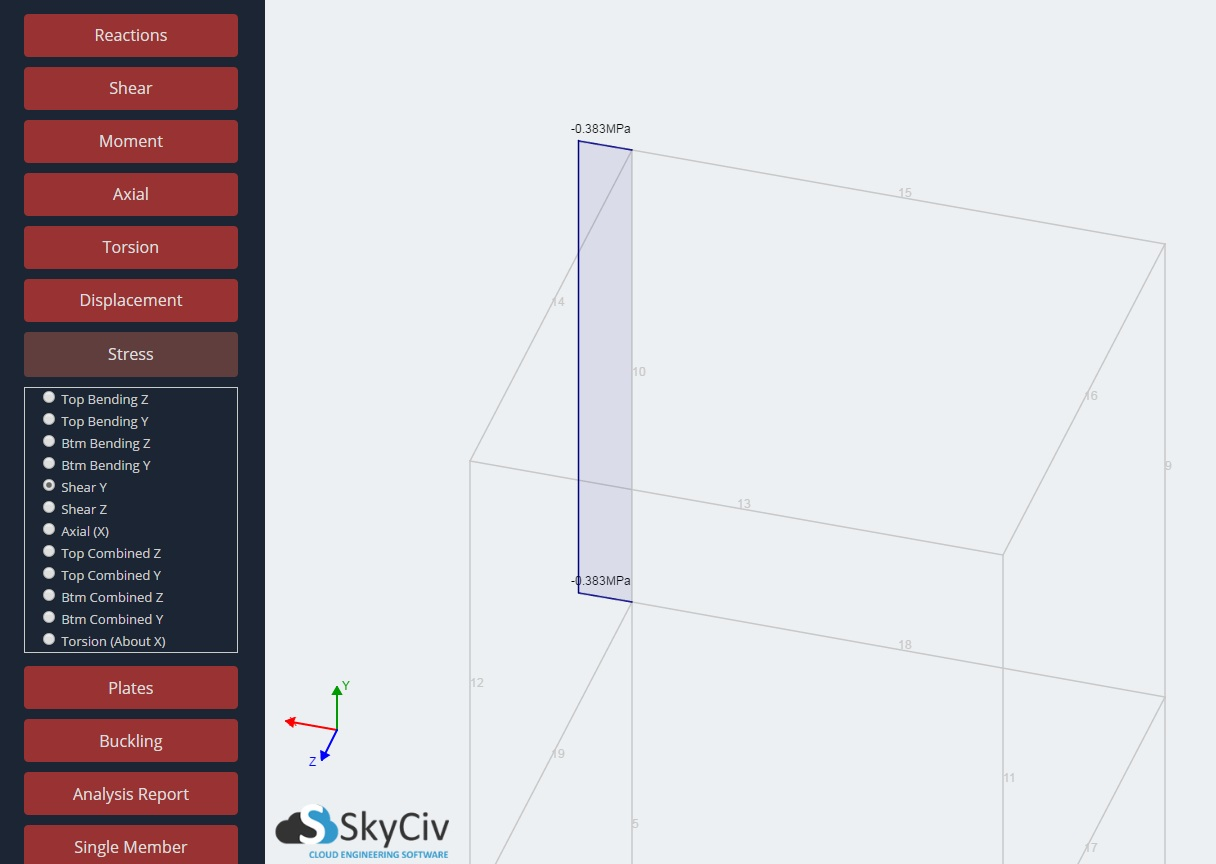## Shear Z

The shear stress in the local Z axis.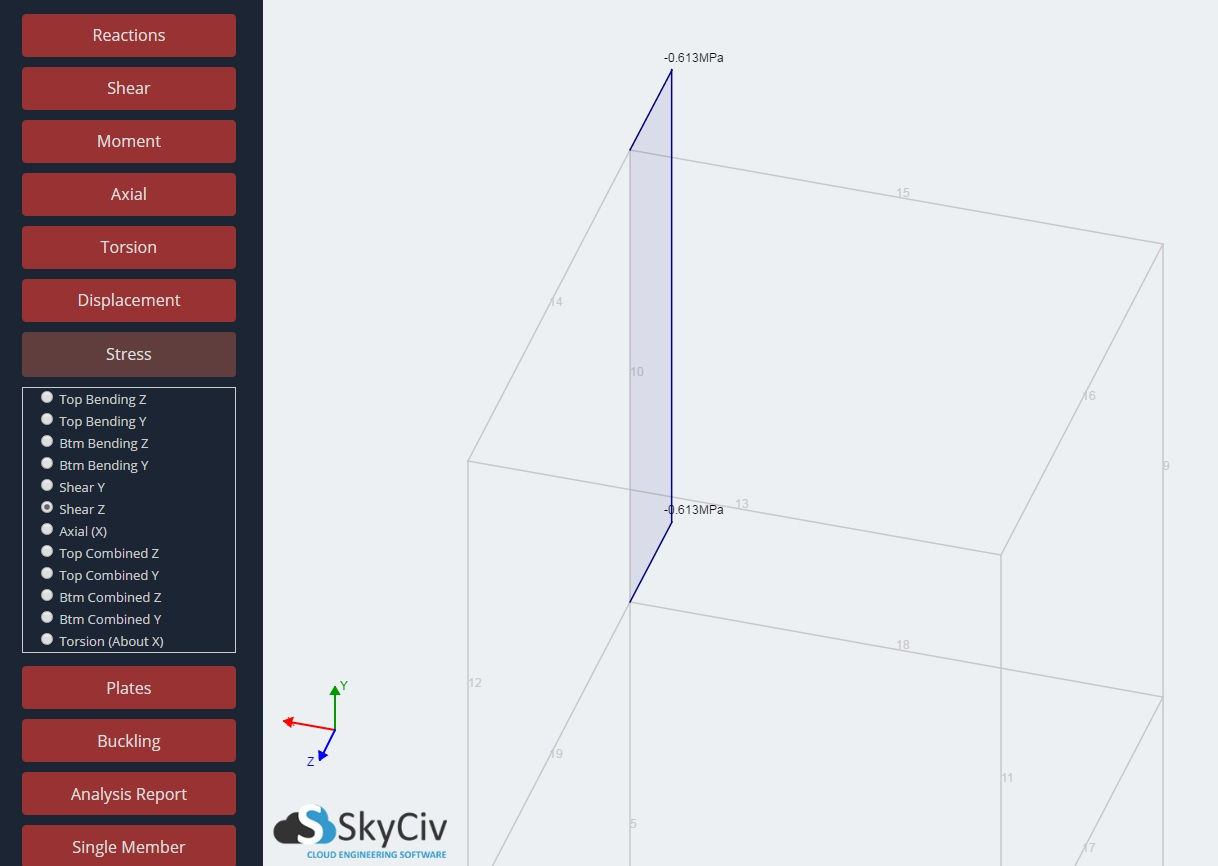## Shear Z

The shear stress in the local Z axis.## Axial (X)

The axial stress in the local X axis.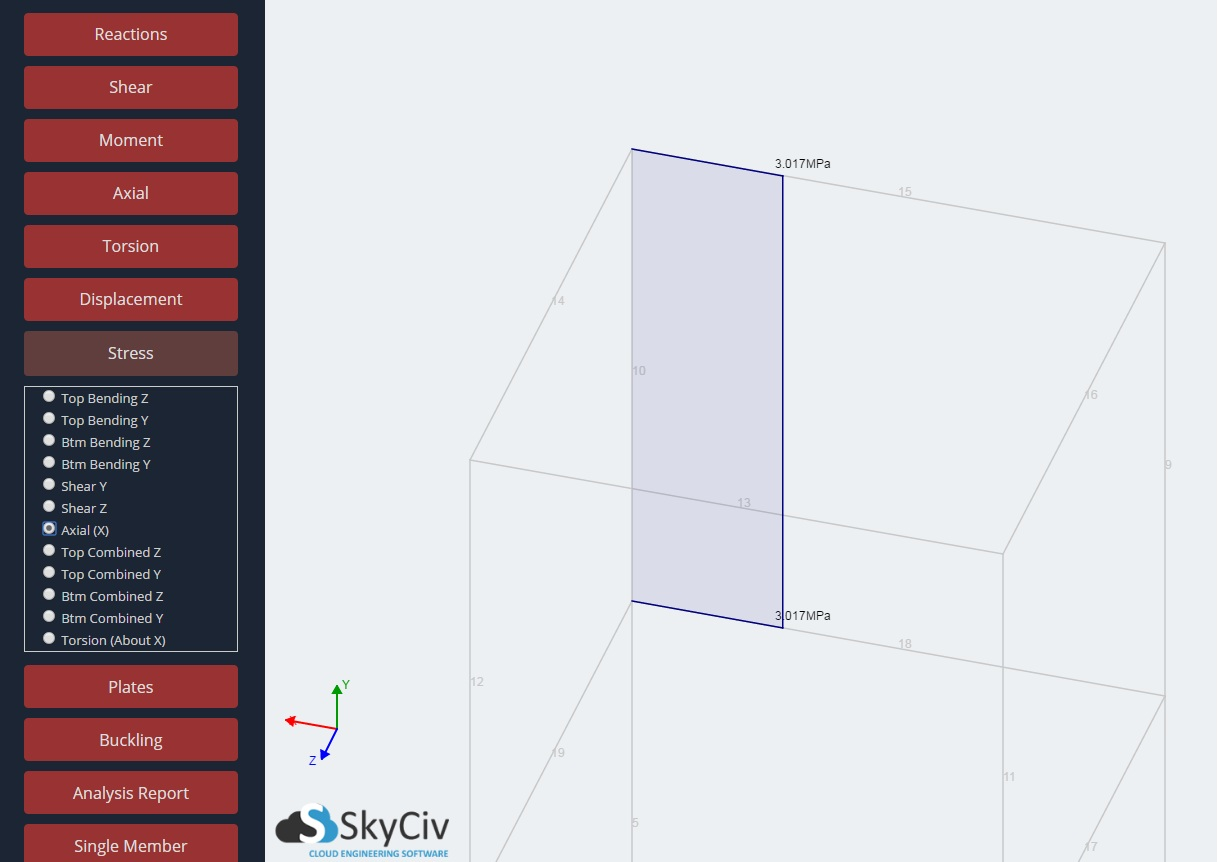## Top Combined Z

The sum of all stresses acting about the Z local axis direction at the top fibre. Sum of Top Bending Z and Axial (X).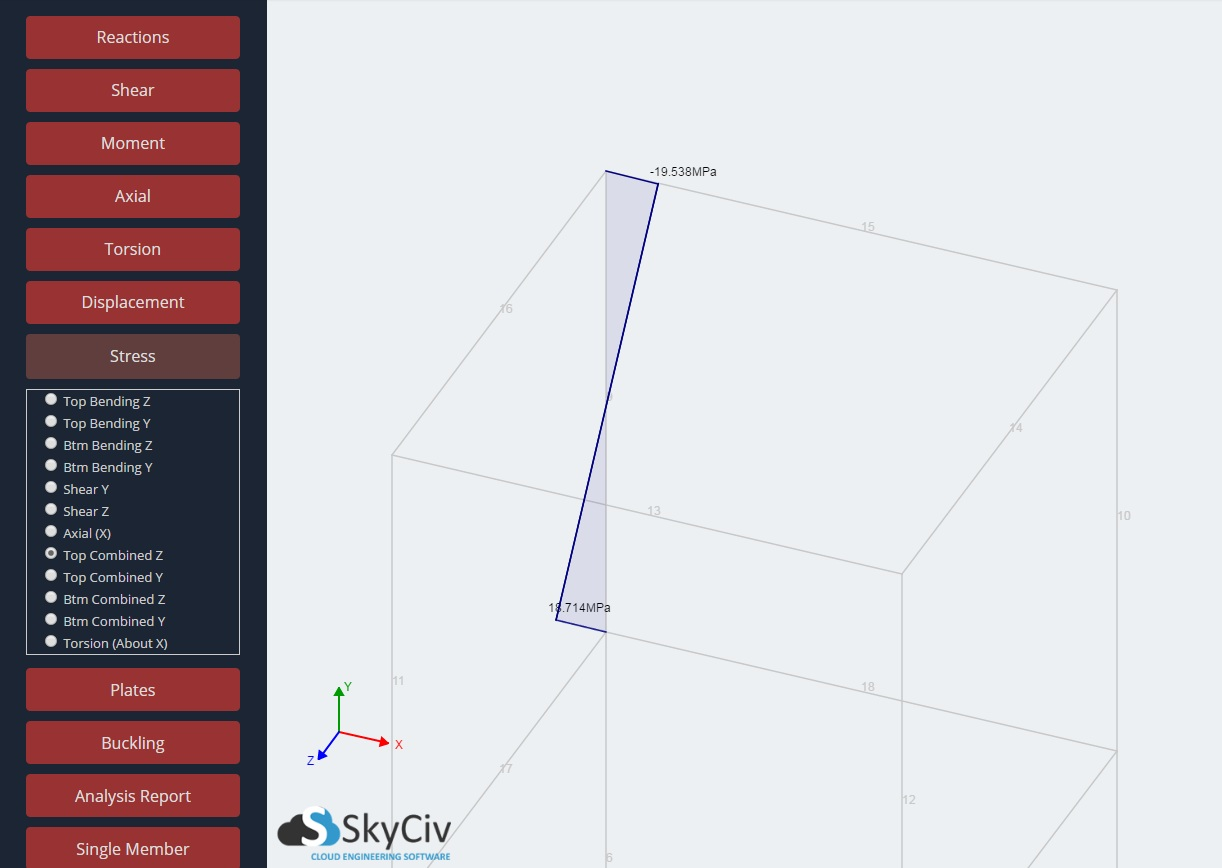## Top Combined Y

The sum of all stresses acting about the Y local axis direction at the top fibre. Sum of Top Bending Y and Axial (X).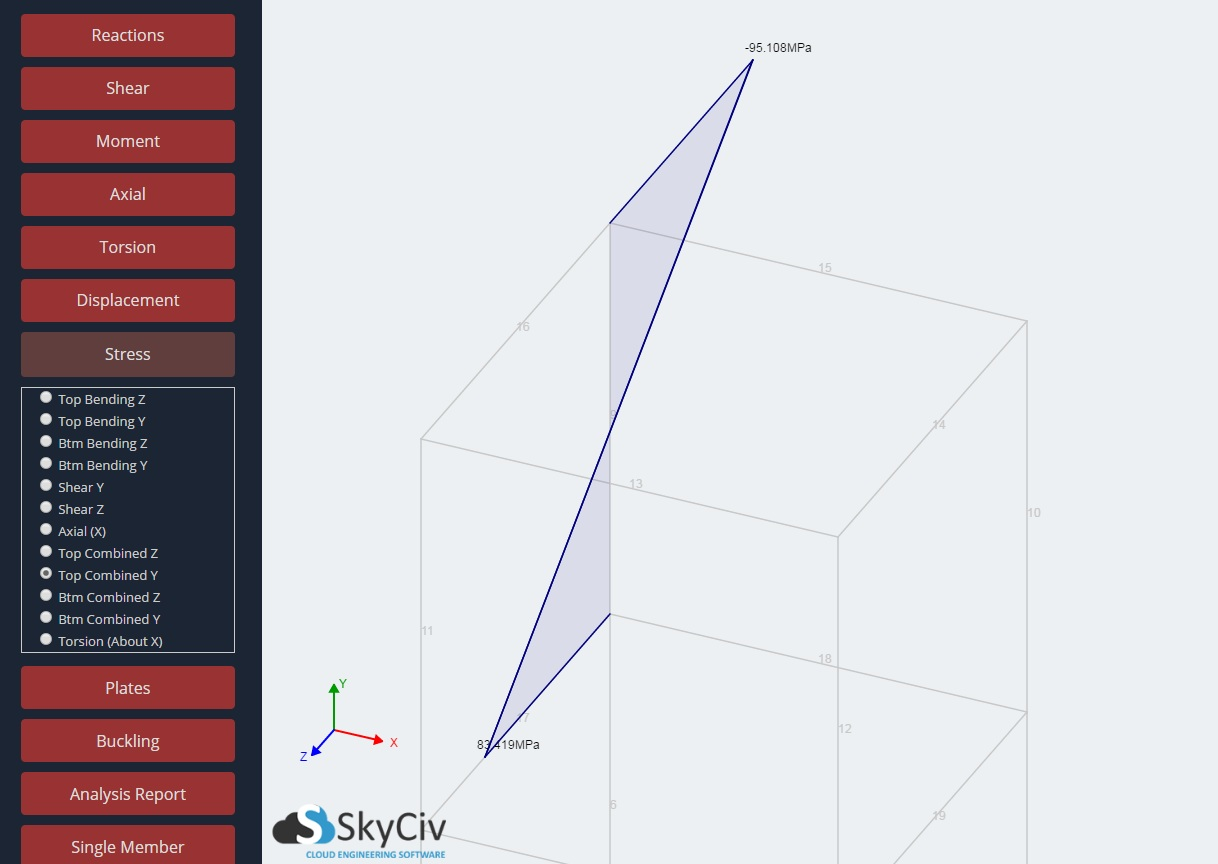## Bottom Combined Z

The sum of all stresses acting about the Z local axis direction at the bottom fibre. Sum of Btm Bending Z and Axial (X).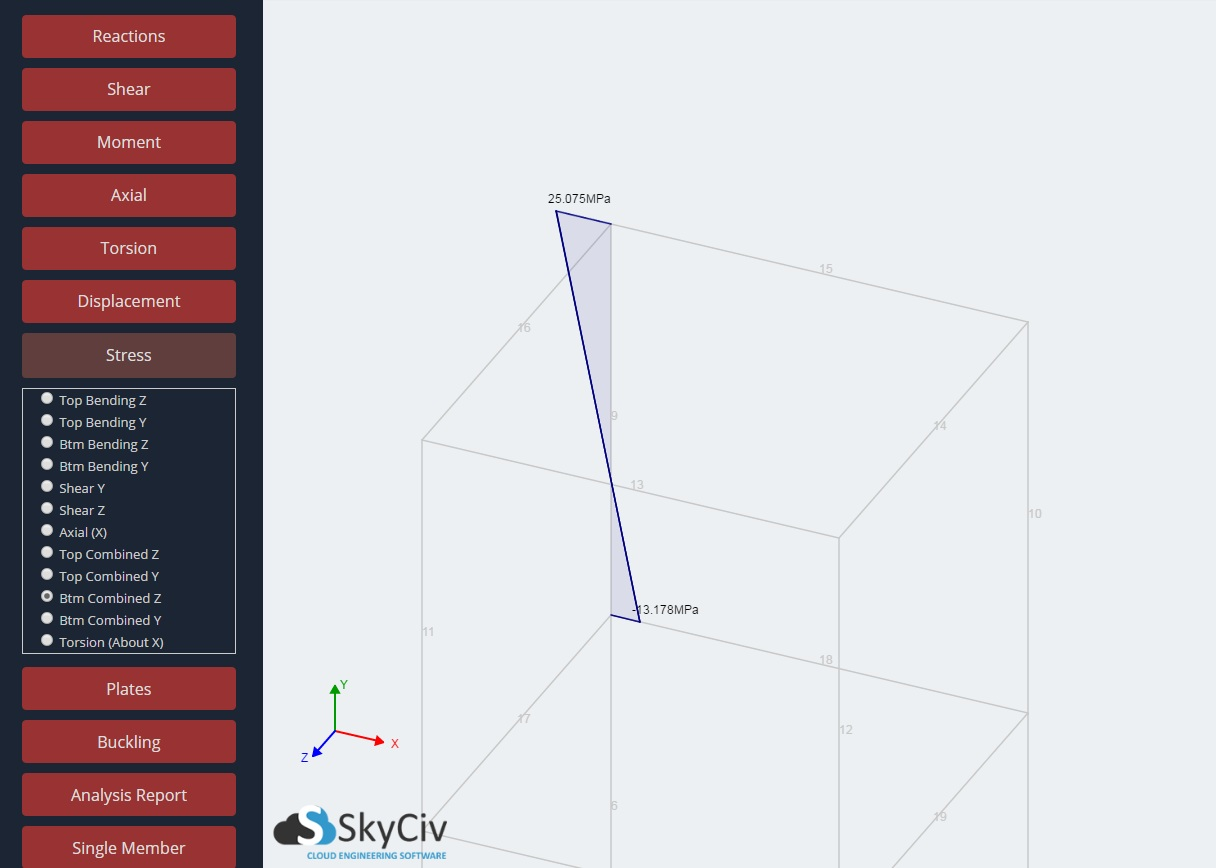## Bottom Combined Y

The sum of all stresses acting about the Y local axis direction at the bottom fibre. Sum of Btm Bending Y and Axial (X).